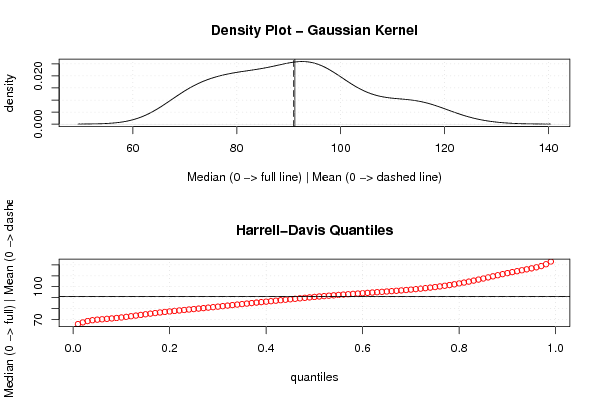## Free Statistics

of Irreproducible Research!

Author's title
Author*Unverified author*
R Software Modulerwasp_meanversusmedian.wasp
Title produced by softwareMean versus Median
Date of computationSun, 06 Jun 2010 19:39:14 +0000
Cite this page as followsStatistical Computations at FreeStatistics.org, Office for Research Development and Education, URL https://freestatistics.org/blog/index.php?v=date/2010/Jun/06/t1275853232427hp1wvij5sf7u.htm/, Retrieved Wed, 31 May 2023 20:11:10 +0000
Statistical Computations at FreeStatistics.org, Office for Research Development and Education, URL https://freestatistics.org/blog/index.php?pk=77781, Retrieved Wed, 31 May 2023 20:11:10 +0000
QR Codes:Original text written by user:
IsPrivate?No (this computation is public)
User-defined keywordsKDGP1W52
Estimated Impact110
Family? (F = Feedback message, R = changed R code, M = changed R Module, P = changed Parameters, D = changed Data)
-     [Notched Boxplots] [Opgave 3 - box pl...] [2010-03-03 16:55:40] [74be16979710d4c4e7c6647856088456]
- RM D    [Mean versus Median] [mediaan - rekenku...] [2010-06-06 19:39:14] [0291ee60c135beb64d296f3dc8feb2dc] [Current]
Feedback Forum

Post a new message
Dataseries X:
93.2
96
95.2
77.1
70.9
64.8
70.1
77.3
79.5
100.6
100.7
107.1
95.9
82.8
83.3
80
80.4
67.5
75.7
71.1
89.3
101.1
105.2
114.1
96.3
84.4
91.2
81.9
80.5
70.4
74.8
75.9
86.3
98.7
100.9
113.8
89.8
84.4
87.2
85.6
72
69.2
77.5
78.1
94.3
97.7
100.2
116.4
97.1
93
96
80.5
76.1
69.9
73.6
92.6
94.2
93.5
108.5
109.4
105.1
92.5
97.1
81.4
79.1
72.1
78.7
87.1
91.4
109.9
116.3
113
100
84.8
94.3
87.1
90.3
72.4
84.9
92.7
92.2
114.9
112.5
118.3
106
91.2
96.6
96.3
88.2
70.2
86.5
88.2
102.8
119.1
119.2
125.1

 Summary of computational transaction Raw Input view raw input (R code) Raw Output view raw output of R engine Computing time 2 seconds R Server 'Sir Ronald Aylmer Fisher' @ 193.190.124.24

\begin{tabular}{lllllllll}
\hline
Summary of computational transaction \tabularnewline
Raw Input & view raw input (R code)  \tabularnewline
Raw Output & view raw output of R engine  \tabularnewline
Computing time & 2 seconds \tabularnewline
R Server & 'Sir Ronald Aylmer Fisher' @ 193.190.124.24 \tabularnewline
\hline
\end{tabular}
%Source: https://freestatistics.org/blog/index.php?pk=77781&T=0

[TABLE]
[ROW][C]Summary of computational transaction[/C][/ROW]
[ROW][C]Raw Input[/C][C]view raw input (R code) [/C][/ROW]
[ROW][C]Raw Output[/C][C]view raw output of R engine [/C][/ROW]
[ROW][C]Computing time[/C][C]2 seconds[/C][/ROW]
[ROW][C]R Server[/C][C]'Sir Ronald Aylmer Fisher' @ 193.190.124.24[/C][/ROW]
[/TABLE]
Source: https://freestatistics.org/blog/index.php?pk=77781&T=0

Globally Unique Identifier (entire table): ba.freestatistics.org/blog/index.php?pk=77781&T=0

As an alternative you can also use a QR Code:

The GUIDs for individual cells are displayed in the table below:

 Summary of computational transaction Raw Input view raw input (R code) Raw Output view raw output of R engine Computing time 2 seconds R Server 'Sir Ronald Aylmer Fisher' @ 193.190.124.24

 Median versus Mean mean 90.9197916666667 median 91.2

\begin{tabular}{lllllllll}
\hline
Median versus Mean \tabularnewline
mean & 90.9197916666667 \tabularnewline
median & 91.2 \tabularnewline
\hline
\end{tabular}
%Source: https://freestatistics.org/blog/index.php?pk=77781&T=1

[TABLE]
[ROW][C]Median versus Mean[/C][/ROW]
[ROW][C]mean[/C][C]90.9197916666667[/C][/ROW]
[ROW][C]median[/C][C]91.2[/C][/ROW]
[/TABLE]
Source: https://freestatistics.org/blog/index.php?pk=77781&T=1

Globally Unique Identifier (entire table): ba.freestatistics.org/blog/index.php?pk=77781&T=1

As an alternative you can also use a QR Code:

The GUIDs for individual cells are displayed in the table below:

 Median versus Mean mean 90.9197916666667 median 91.2PNG link Postscript link PDF link

Parameters (Session):
par1 = grey ;
Parameters (R input):
R code (references can be found in the software module):
library(Hmisc)m <- mean(x)e <- median(x)bitmap(file='test1.png')op <- par(mfrow=c(2,1))mydensity1 <- density(x,kernel='gaussian',na.rm=TRUE)plot(mydensity1,main='Density Plot - Gaussian Kernel',xlab='Median (0 -> full line) | Mean (0 -> dashed line)',ylab='density')abline(v=e,lty=1)abline(v=m,lty=5)grid()myseq <- seq(0.01, 0.99, 0.01)hd <- hdquantile(x, probs = myseq, se = TRUE, na.rm = FALSE, names = TRUE, weights=FALSE)plot(myseq,hd,col=2,main='Harrell-Davis Quantiles',xlab='quantiles',ylab='Median (0 -> full) | Mean (0 -> dashed)')abline(h=m,lty=5)abline(h=e,lty=1)grid()par(op)dev.off()load(file='createtable')a<-table.start()a<-table.row.start(a)a<-table.element(a,'Median versus Mean',2,TRUE)a<-table.row.end(a)a<-table.row.start(a)a<-table.element(a,'mean',header=TRUE)a<-table.element(a,mean(x))a<-table.row.end(a)a<-table.row.start(a)a<-table.element(a,'median',header=TRUE)a<-table.element(a,median(x))a<-table.row.end(a)a<-table.end(a)table.save(a,file='mytable.tab')